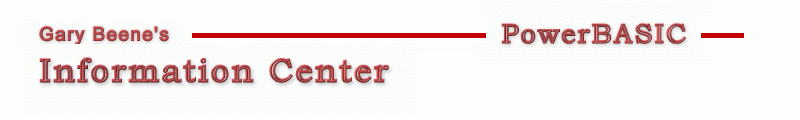## Focus Rectangle - Over Dialog, Persistent, Manually Draw Points

Category: Drawing

Date: 03-28-2012

'This code allows drawing a rectangle on the dialog by using the mouse.

'This code is written to compile in PBWin10. To compile in PBWin9, split pt
'into pt.x and pt.y as arguments wherever the PtinRect() API is used (2 places).

'Compilable Example:
#Compiler PBWin 10
#Compile EXE
#Dim All
#Include "Win32API.inc"
Global hDlg, hDC As Dword, R, R2 As Rect, pt As Point, ddx,ddy,w,h As Long

Function PBMain() As Long
Dialog New Pixels, 0, "Draw Rectangle",300,300,420,250, %WS_OverlappedWindow To hDlg
Dialog Show Modal hDlg Call DlgProc
End Function

CallBack Function DlgProc() As Long
Select Case Cb.Msg
Case %WM_RButtonDown
pt.x = Lo(Word,Cb.LParam)
pt.y = Hi(Word,Cb.LParam)
If PtinRect(R,pt) Then
w = R.nRight - R.nLeft
h = R.nBottom - R.nTop
ddx = R.nLeft - pt.x
ddy = R.nTop -  pt.y
Else
DrawRect 0 : Reset R
End If
Case %WM_LButtonDown
R.nLeft = Lo(Word, Cb.LParam)   : R.nTop  = Hi(Word, Cb.LParam)
Case %WM_MouseMove
pt.x = Lo(Word,Cb.LParam)
pt.y = Hi(Word,Cb.LParam)
If (Cb.WParam And %MK_LBUTTON) Then
R.nRight  = pt.x : R.nBottom = pt.y
DrawRect 1
ElseIf (Cb.WParam And %MK_RButton) And PtinRect(R,pt) Then
DrawRect 0
R.nLeft = pt.x + ddx : R.nRight = R.nLeft + w
R.nTop  = pt.y + ddy : R.nBottom = R.nTop + h
DrawRect 1
End If
End Select
End Function

Sub DrawRect(Flag As Long)   '1=moving 0=done
Local i As Long, tempR As Rect
hDC = GetDC(hDlg)
DrawFocusRect hDC, R2
tempR.nLeft = Min(R.nLeft,R.nRight) : tempR.nRight = Max(R.nLeft,R.nRight)
tempR.nTop = Min(R.nTop,R.nBottom)  : tempR.nBottom = Max(R.nTop,R.nBottom)
If Flag Then DrawFocusRect hDC, tempR : R2 = tempR Else Reset R2
ReleaseDC hDlg, hDC
End Sub

'gbs_00875
'Date: 03-10-2012

created by gbSnippets
http://www.garybeene.com/sw/gbsnippets.htm